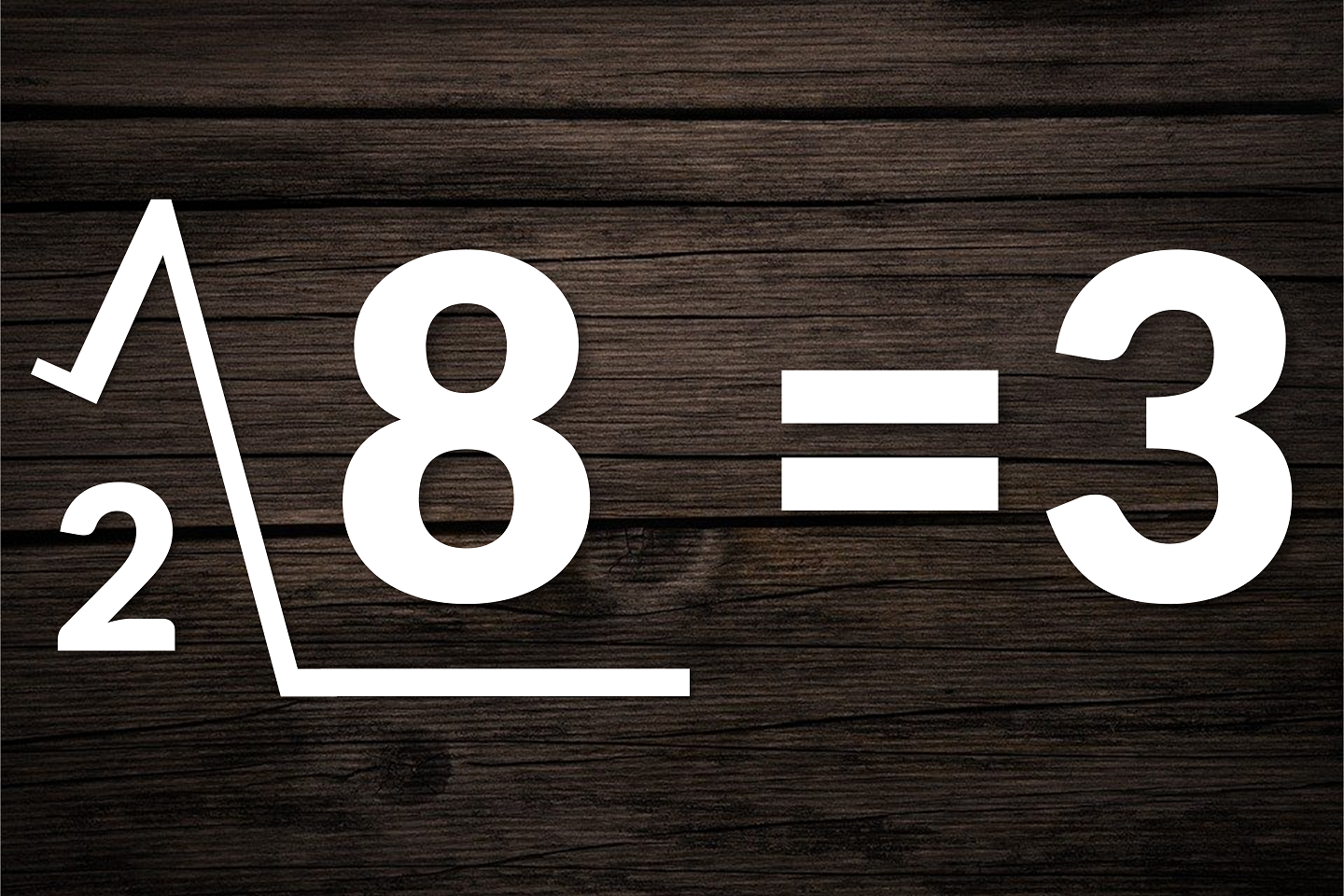# A Radical New Look for Logarithms

April 24, 2015
Mathematics

"A good notation has a subtlety and suggestiveness which at times make it almost seem like a live teacher." — Bertrand Russell

"We could, of course, use any notation we want; do not laugh at notations; invent them, they are powerful. In fact, mathematics is, to a large extent, invention of better notations." — Richard Feynman

"By relieving the brain of all unnecessary work, a good notation sets it free to concentrate on more advanced problems, and in effect increases the mental power of the race." — Alfred North Whitehead

Notation is perhaps one of the most important aspects of mathematics.  The right choice of notation can make a concept clear as day; the wrong choice can make extracting its meaning hopeless.  Of course, one great thing about notation is that even if there's a poor choice of notation out there (such as $$\left[x\right]$$ or $$\pi$$), often someone comes along and creates a better one (such as $$\lfloor x\rfloor$$ for the floor function or multiples of tau, $$\tau\approx 6.28318$$, for radian measure of angles).

Which brings me to one such poor choice of notation, one that I believe needs fixing:  the rather asymmetrical notation of powers, roots, and logarithms.

Here we have three very closely related concepts — both roots and logarithms are ways to invert exponentiation, the former returning the base and the latter returning the exponent.  And yet their notation couldn't be more different:
\begin{align*} 2^3 &= 8\\ \sqrt{8} &= 2\\ \log_2{8}&=3 \end{align*}This always struck me as annoyingly inelegant.  Wouldn't it be nice if these notations bore at least some resemblance to each other?

After giving it some thought, I believe I have found a possible solution.  As an alternative to writing $$\log_2 {8}$$, I propose the following notation:

This notation makes use of a reflected radical symbol, such that the base of the logarithm is written in a similar manner to the index of a radical but below the "point" (the pointy part of the radical symbol), and the argument of the logarithm is written "inside".  The use of this notation has a number of advantages:

1. The symmetry between the normal radical for roots and the reflected radical for logarithms highlights both their similarities and their differences — each one "undoes" an exponential expression, but each one gives a different part of the expression (the base and the exponent, respectively.)
2. The radical symbol can be looked at as a modified lowercase letter "r".  (This may actually be the origin of the symbol, where the "r" stands for radix, the Latin word for "root".)  In a similar way, the new symbol for logarithms resembles a capital "L".
3. The placement of the "small number" and the "point" can take on a secondary spatial meaning:
• The "small number" represents a piece of information we know about an exponential expression, and its placement indicates which part we know.
--- For a root, the "small number" is on top, so we know the exponent.
--- For a logarithm, the "small number" is on bottom, so we know the base.
• The symbol seems to "point" to the piece of information that we are looking for.
--- For a root, the "point" is pointing downward, so we are looking for the base.
--- For a logarithm, the "point" is pointing upward, so we are looking for the exponent.
• Looking at the image above, the new notation seems to say "We know the base is 2, so what's the exponent that will get us to 8?"
• Similarly, the expression $$\sqrt{8}$$ now can be interpreted as saying "We know the exponent is 3, so what's the base that will get us to 8?"

This notation would obviously not make much of a difference for seasoned mathematicians who are perfectly comfortable with the $$\log$$ and $$\ln$$ functions.  But from a pedagogical standpoint, the reflected radical, with its multi-layered meaning and auto-mnemonic properties, could help students become more comfortable with a concept that many look at as just meaningless manipulation of symbols.

When I first came up with this reflected-radical notation, I had originally imagined that it should replace the current notation.  However, after some feedback from various people and some further consideration, I think a better course of action would be to have this notation be used alongside the current notation, much in the way that we have multiple notations for other concepts in math (such as the many ways to write derivatives).  However, I would suggest that, if it were to become commonplace*, this notation would be best to use when first introducing the concept in schools.  The current notation isn't wrong per se — it's just not very evocative of the underlying concept.  Anything that can better elucidate that concept can't be a bad thing when it comes to students learning mathematics!

It may seem like a radical idea.

But it's a logical one.

* Of course, for this notation to become commonplace, somebody would need to figure out how to replicate it in LaTeX.  Any takers?